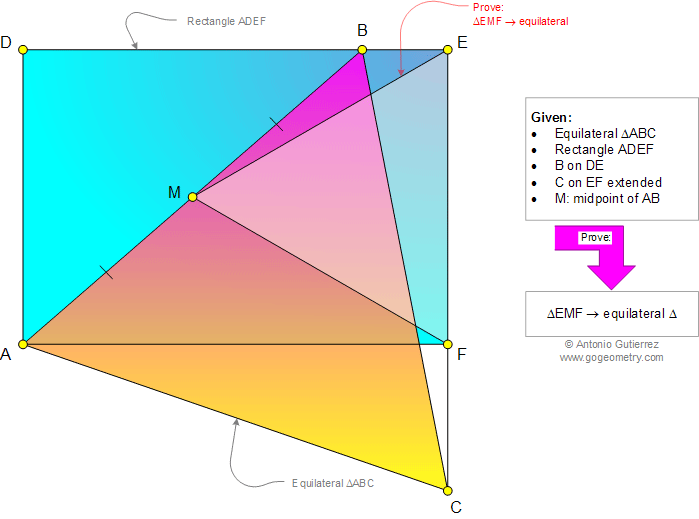# Geometry Problem 968: Equilateral Triangle, Rectangle, Common Vertex, Midpoint. Level: School, College, Mathematics Education

< PREVIOUS PROBLEM  |  NEXT PROBLEM >

 The figure shows a rectangle ADEF and equilateral triangle ABC (B on DE, C on EF extended). If M is the midpoint of AB, prove that triangle EMF is equilateral.Home | Search | Geometry | Problems | All Problems | Open Problems | Visual Index | 10 Problems | Problems Art Gallery |  Art | 961-970 | Triangles | Equilateral Triangle | Rectangle | Midpoint | by Antonio Gutierrez